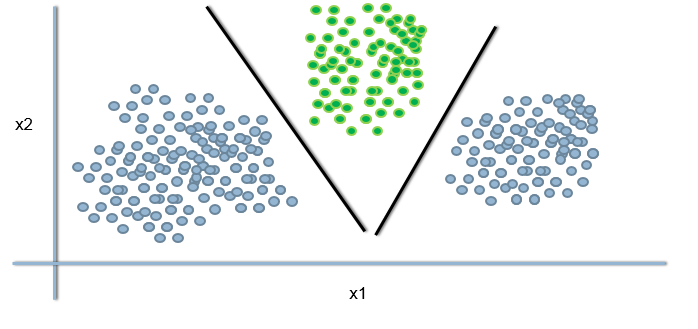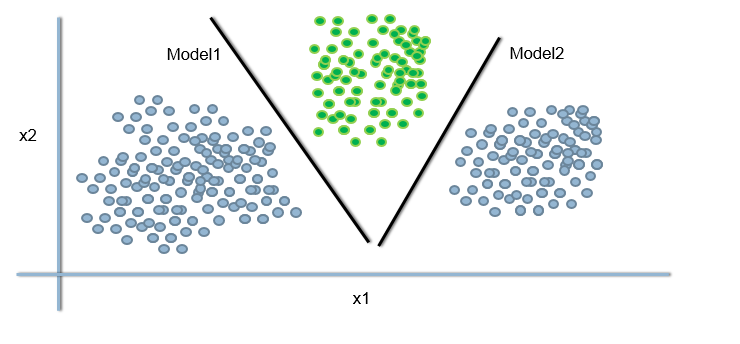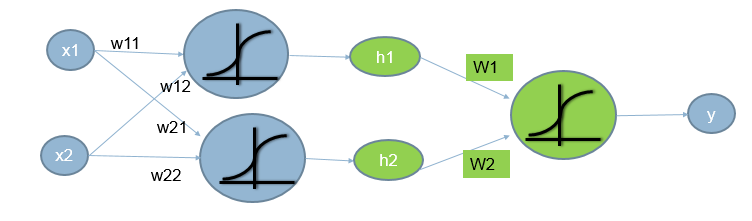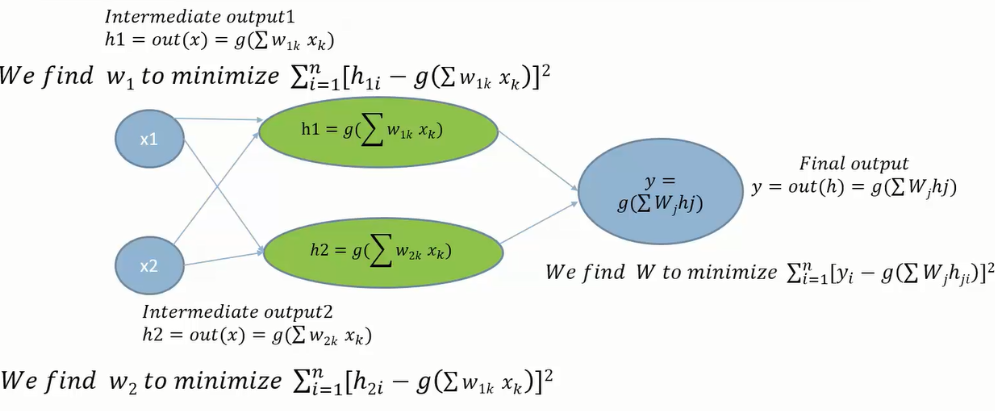• No products in the cart.

# 204.5.4 Issue with Non Linear Decision Boundary

##### Understanding intermediate outputs.
Link to the previous post : https://statinfer.com/204-5-3-practice-non-linear-decision-boundary/

In the  previous post we just tried solving a non linear data using linear boundary. We can use multiple linear boundaries to separate classes in this kind of datasets.

However, this will cause some issues which we will understand but visualizing the problem and think about a possible solution too.

### Non-Linear Decision Boundaries-Issue

Logistic Regression line doesn’t seem to be a good option when we have non-linear decision boundaries.We can try to build two different models and have two difference linear decision boundaries. These two models will give us intermediate outputs and combining both will give us the final output.

### Non-Linear Decision Boundaries-SolutionIntermediate Output1 Intermediate Output2
out(x)= $$g(\sum w_kx_k)$$ say h1 out(x)= $$g(\sum w_kx_k)$$ say h2

#### The Intermediate output

• Using the x’s directly; predicting y is challenging.
• We can predict h, the intermediate output, which will indeed predict Y#### Finding the Weights for Intermediate OutputsIf we increase the number of intermediate outputs and add a few layers of intermediate models, we end up with a basic neural network.
The next post is a practice session on implementing intermediate outputs in python.
Link to the next post  :  https://statinfer.com/204-5-5-practice-implementing-intermediate-outputs-in-python/
0 responses on "204.5.4 Issue with Non Linear Decision Boundary"